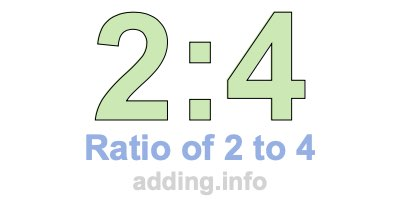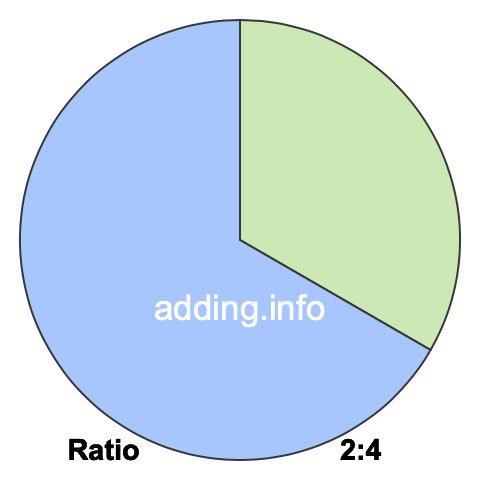Ratio of 2 to 4A ratio of 2 to 4 can be written as 2 to 4, 2:4, or 2/4. Furthermore, 2 and 4 can be the quantity or measurement of anything, such as students, fruit, weights, heights, speed and so on.

A ratio of 2 to 4 simply means that for every 2 of something, there are 4 of something else, with a total of 6.

In the box below, you can enter a number in either box and we will calculate the other number to keep the ratio of 2 to 4. In other words, we will calculate the corresponding equivalent ratio of 2:4 for you.

:

To calculate the left side of the ratio, our calculator multiplies the right side by 2 and then divides the product by 4. To calculate the right side of the ratio, our calculator multiplies the left side by 4 and then divides the product by 2. Answers are rounded to two decimals if necessary.

Below is a list of equivalent ratios of 2:4 that we created using our 2:4 ratio calculator above.

1:2

2:4

3:6

4:8

5:10

6:12

7:14

8:16

9:18

10:20

11:22

12:24

We have also created a pie chart for you to put 2:4 ratio in perspective. To do so, we divided a pie into 6 pieces and then colored 2 pieces green and 4 pieces blue. The pie will look exactly the same using any equivalent ratio of 2:4.The green part of the pie covers 33.33% and the blue part covers 66.67%. To calculate the percentages, we divided each side of the ratio by its total and then multiplied by 100, like this:

(2 / 6)×100 ≈ 33.33
(4 / 6)×100 ≈ 66.67

In fractional terms, 2/4 of the pie above is colored in green. This means that the ratio of 2 to 4 or 2:4 can also be expressed as a fraction, where the 2 to the left of the colon is the numerator and the 4 to the right of the colon is the denominator:

2:4 = 2/4

Ratio is often used when defining rectangles in width:height format. Width and height can be feet, inches, centimeters, or any other kind of length. With a ratio of 2 to 4, the width is 2 and the height is 4. Below is a picture of what a rectangle with a ratio of 2:4 looks like.Since the simplest form of the fraction 2/4 is 1/2, the simplest form of the ratio 2:4 is also 1:2.

Ratio Calculator
Need information about another ratio? Here you can submit another ratio for us to explain.

:

Ratio of 2 to 5
Go to the next ratio on our list that we have detailed information about.